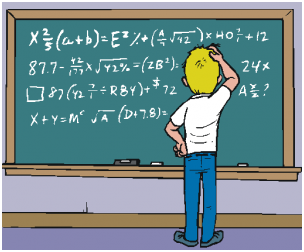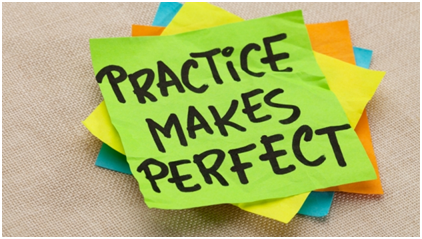# How to master JEE Main Mathematics Syllabus

Mathematics is a dreaded subject for many students but certainly is not an impossible dream. In JEE, you get 30 questions in the Mathematics section which is same as Physics and Chemistry. The level of difficulty of the entire set is not different than the other two subjects.

Created On: Feb 23, 2017 10:49 ISTHow to master JEE Main Mathematics Syllabus

Mathematics is a dreaded subject for many students but certainly is not an impossible dream. In JEE,you get 30 questions in the Mathematics section which is same as Physics and Chemistry. The level of difficulty of the entire set is not different than the other two subjects.

If you want to score good marks in Mathematics section, you would need to start your preparation early. The last minute cramming does not work with Mathematics. But, if you have dedicatedly prepared for the subject, there is no need to worry.

The Main Mantra

The main mantra of mastering Mathematics as a subject is to know all formulae well. What remains is just the application of these concepts.

You can jot down list of all formulae and main concepts in the form of notes somewhere. You can always understand how the formulae were arrived at so that learning them makes it easier to remember. You must learn all the formulae before the exam and applying them again and again will make you remember them forever.

Once formulae and main concepts are clear in your mind, proceed to questions. A question may be direct or application based. For a direct question, you can apply a formula directly. If all the inputs to a formula are given in the question the question is very easy. Otherwise, jot down the unknowns and figure out a way to find them out. This process can also be straightforward or complex but the hints for finding out the unknowns are always given in the question. In a straightforward question, the information you need is in the same chapter from which the question is asked. In a complicated question, you may need to combine concepts from several chapters then arrive at all the unknowns. Such questions can be prepared in the end when you have completed all the chapters.

Sometimes a substitution may be required to bring the question into a form where it matches the formulae provided. This requires a good observation and a memory of all such tricks used in the questions you have practiced. So solve first 20 questions on each chapter with formulae and notes sheet open. You should make changes to your notes as you learn new tricks. Then start solving questions without any reference. You should refer back to formulae and notes sheets at regular intervals.How to approach Mathematics Syllabus

Mathematics in JEE is divided in primarily sections: Algebra and Arithmetic, Geometry, Calculus and Probability.

Master Algebra and Arithmetic first. After mastering Algebra and Arithmetic, you must start with Geometry and followed by Trigonometry. It is a more visual concept and it always helps to make a diagram side by side to a question, annotate it with values given in the question, apply concepts learnt to the question and then arrive at the answer. Trigonometry becomes easy, if your Algebra and basic geometry both are strong.

After this, Calculus should be done because it requires that you are comfortable with Algebra, Geometry and Trigonometry. Probability and other topics can be taken up in the end or whenever you are comfortable but the main idea of solving the questions remains same.

Some JEE Tricks

In Mathematics, the age old saying “Practice makes a man perfect” holds very well. The more questions you practice the more tricks you will learn and remember. You will also be able to solve questions faster if you practice more. Specifically for JEE Main, practice doing calculations in mind and learn tricks to do simple calculations faster. This will help you save time. You can attempt the Mathematics section in the end because it will take the maximum time. Also, in the exam, you must attempt the questions first on which you are fully confident.Mathematics is not a difficult subject but certainly there is no shortcut to master it. So, start early and practice regularly.

ALL THE BEST

Author Name: Yogita Bhalla

Description: Yogita Bhalla is Founder and CEO of Simplylearnt.com and has a passion for helping students excel in their lives. She has appeared for 6 different competitive exams (including NTSE, IIT-JEE, GRE) and has gone through the toiling preparation process. She believes that study material can be made more engaging for the students and hence they can fall in love with test preparation.

Comment ()

7 + 3 =
Post